Constructing Quadrilateral - 4 sides and diagonal given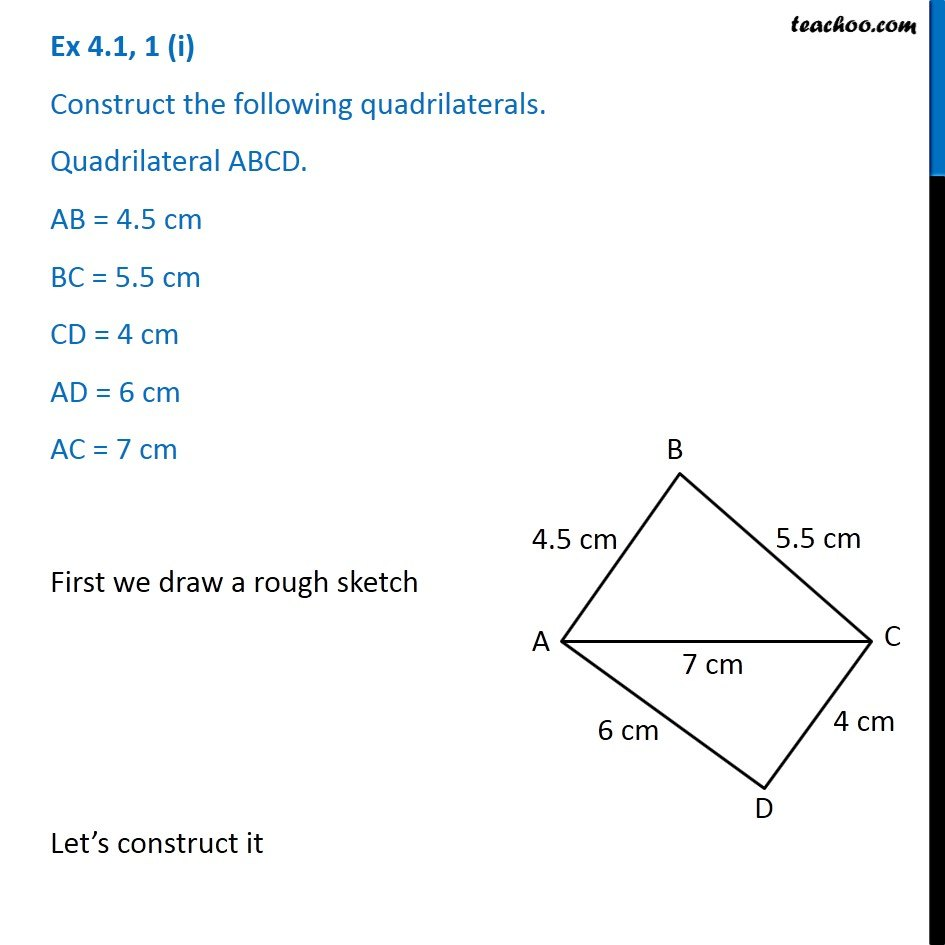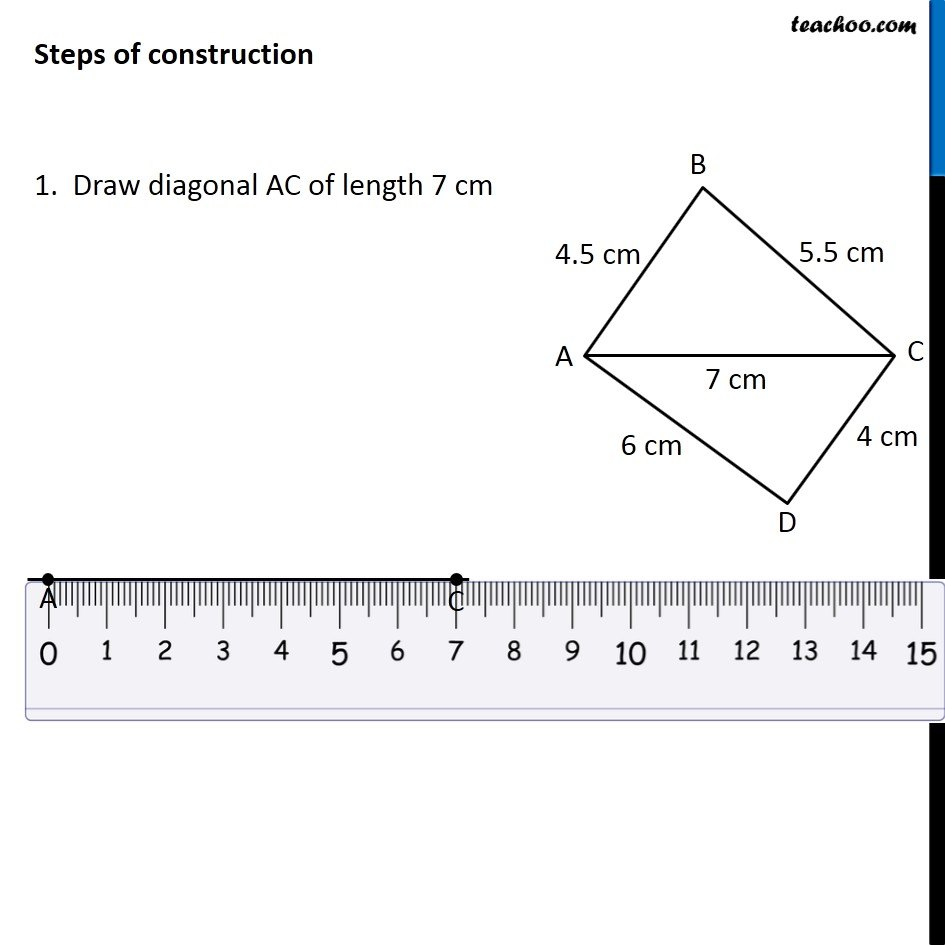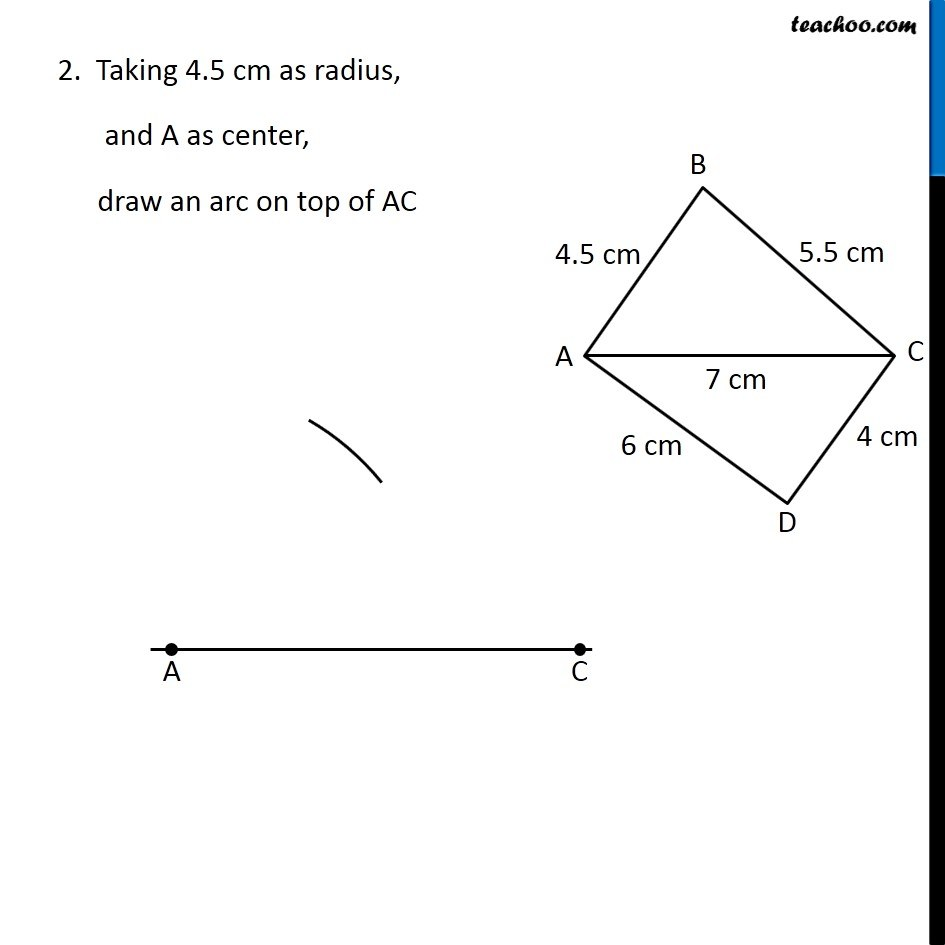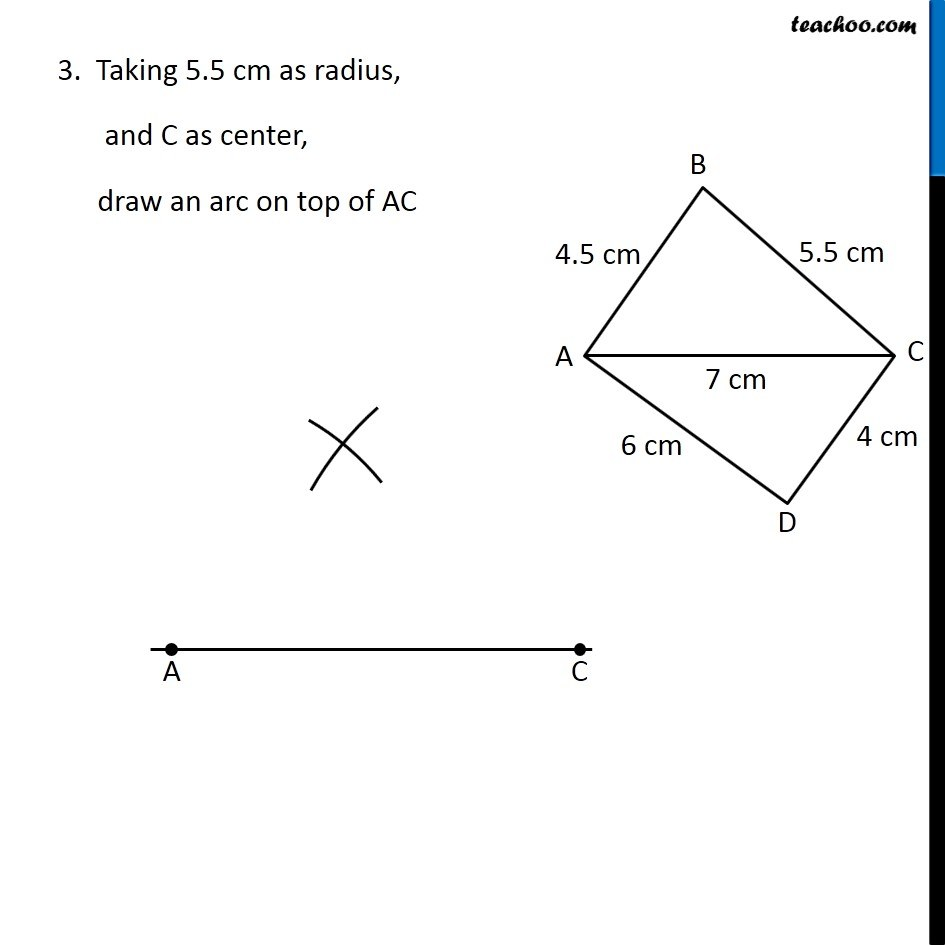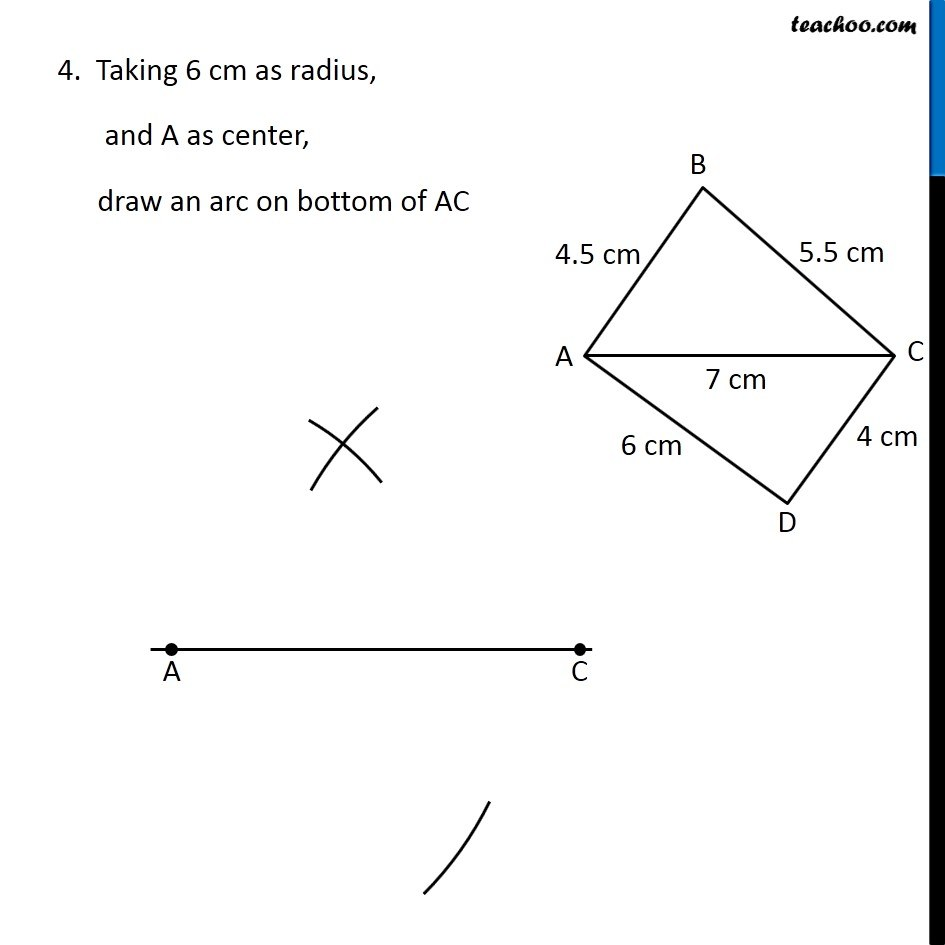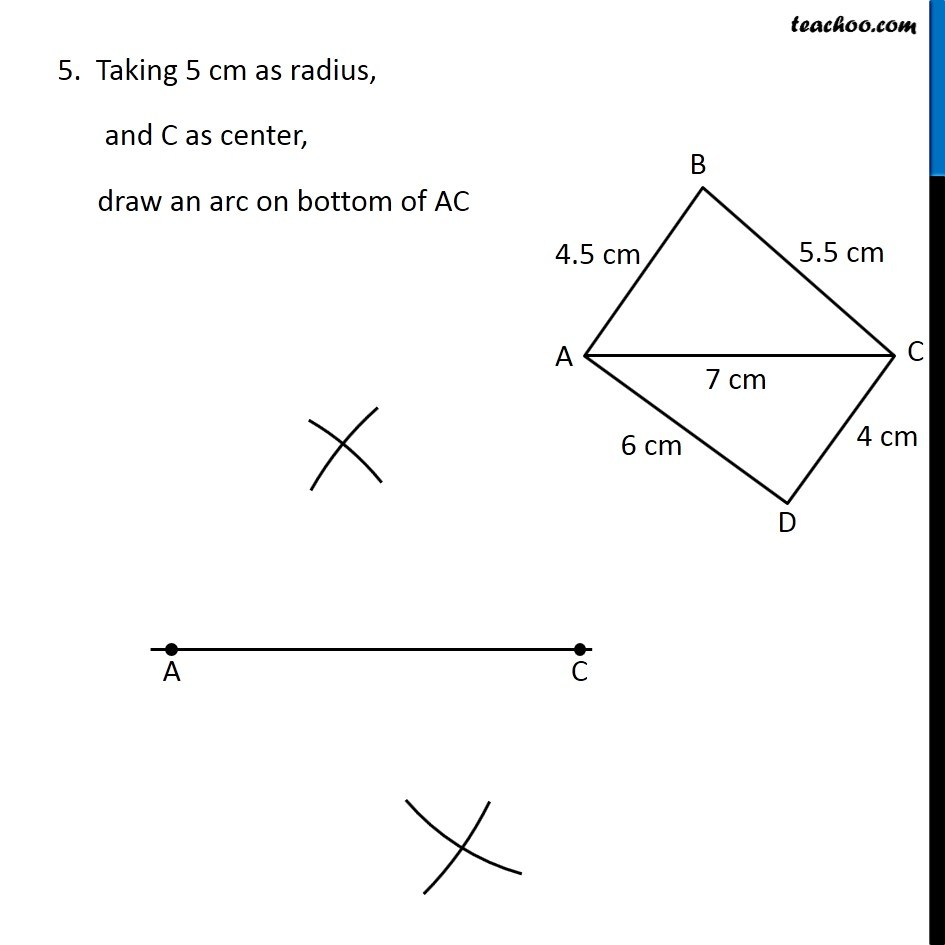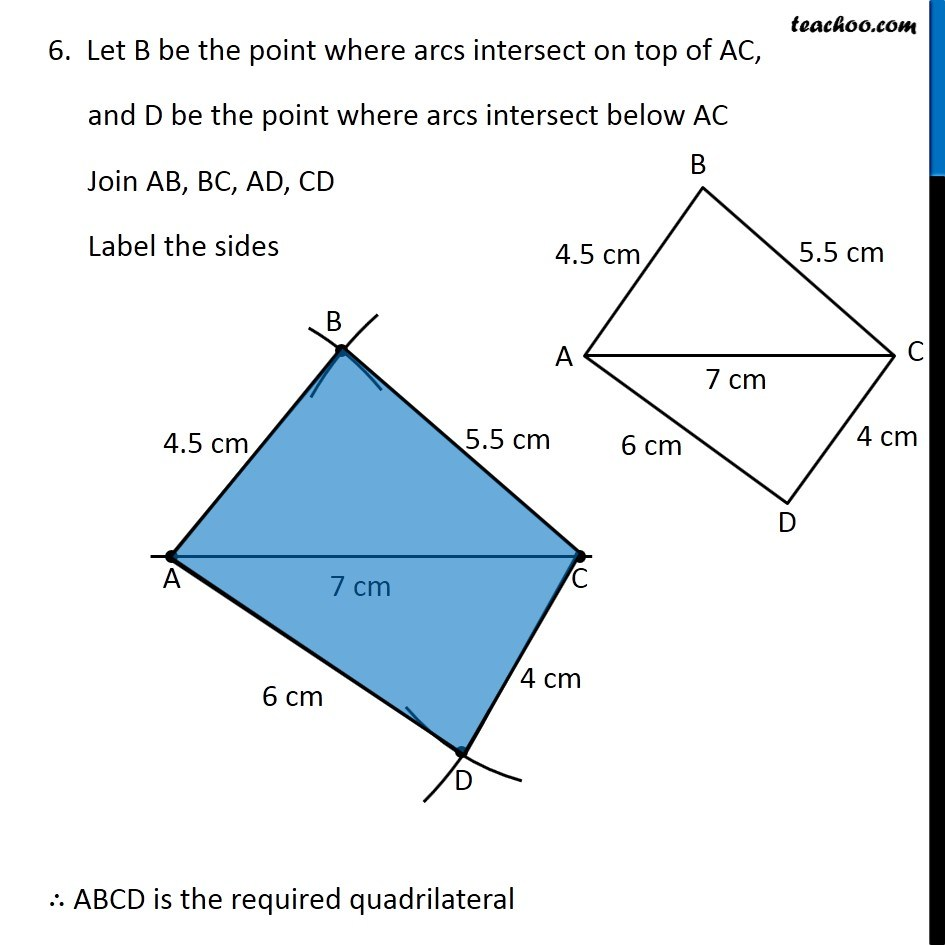Learn in your speed, with individual attention - Teachoo Maths 1-on-1 Class

### Transcript

Question 1 (i) Construct the following quadrilaterals. Quadrilateral ABCD. AB = 4.5 cm BC = 5.5 cm CD = 4 cm AD = 6 cm AC = 7 cm First we draw a rough sketch Let’s construct it Steps of construction 1. Draw diagonal AC of length 7 cm 2. Taking 4.5 cm as radius, and A as center, draw an arc on top of AC 3. Taking 5.5 cm as radius, and C as center, draw an arc on top of AC 4. Taking 6 cm as radius, and A as center, draw an arc on bottom of AC 5. Taking 5 cm as radius, and C as center, draw an arc on bottom of AC 6. Let B be the point where arcs intersect on top of AC, and D be the point where arcs intersect below AC Join AB, BC, AD, CD Label the sides ∴ ABCD is the required quadrilateral